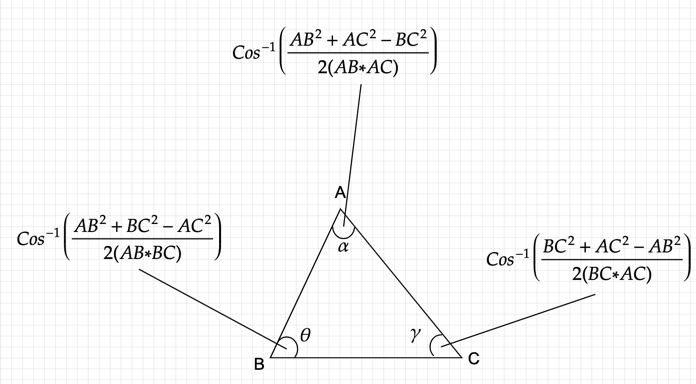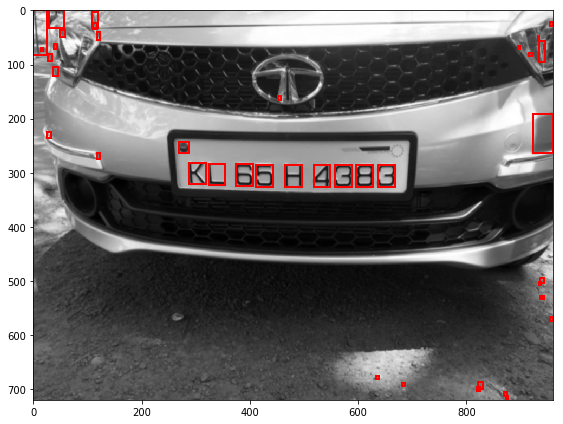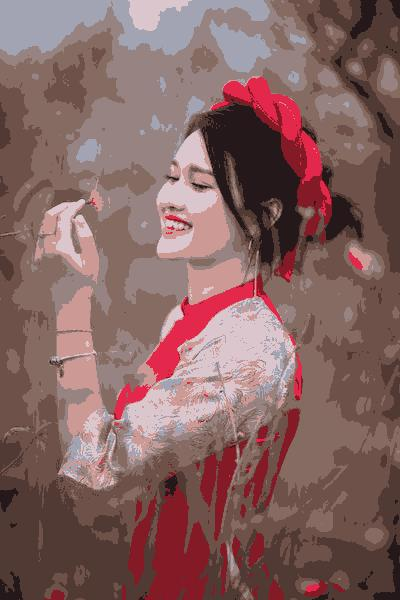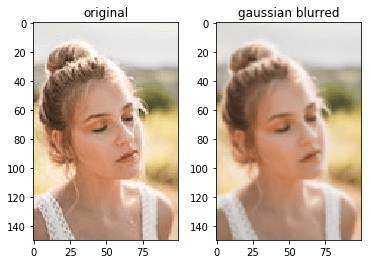## Using the law of cosines and vector dot product formula to find the angle between three points

For any 3 points A, B and C on a cartesian plane. If we have to find the angle between these points, there are many ways we can do that. In this article I will talk about the two frequently used methods: The Law of Cosines formula Vector Dot product formula Law of Cosines For …## Algorithm for detecting and extracting number plates from images of cars

Abstract This article presents a method for automatic detection and extraction of number plates from the images of cars. There are usually three steps in an Automatic Number Plate Recognition (ANPR) system. The first one is to binarize the image and separate the background from the foreground. The Foreground contains the numbers of the number …## Reduce the number of Colors of an image using K-Means Clustering

This article presents a method for reducing the number of colors in an image using K-means clustering. This is a continuation of my previously posted color quantization using Uniform Quantization and Median Cut Quantization. K-Means is one of the simplest unsupervised clustering algorithm used to cluster data into K clusters. The algorithm iteratively assigns the …## Reducing the number of colors of an image using Median Cut algorithm

In my previous post, I talked about the Uniform Quantization algorithm, which was a simple way of reducing the colors in the image. Though it’s easy to implement, it doesn’t always yield good results when there are many colors belonging to the same region. Also, a lot of color regions will not have any colors …## Reduce the number of Colors of an image using Uniform Quantization

Reducing the number of colors in an image is also called Color quantization. It’s commonly used for generating GIF images which currently supports only 256 colors. The general idea is, group similar colors in an image into regions, replace them with the color which closely resembles or represents the region. This color is also called …

## Why isn’t n/0 allowed in mathematics.

If you divide 4 by 2 you would get 2 as the quotient, which means there are exactly two 2’s in 4. And similarly, If you divide 5 by 2, you would get 2 as the quotient and 1as the remainder, which means there are two 2’s in 5 and a 1 which if you …## Basics of Image Convolution

Convolution is a process used for applying general-purpose filter effects like blurring, sharpening, embossing, edge detection, and more. To understand convolutions we must first understand what a convolution matrix is, also referred to as kernel. Take for example the blurring filter. In blur filter, we set each pixel to the mean of its neighbouring pixels. …## Segmenting lines in handwritten documents using A* Path planning algorithm

In this article, I will explain a widely used method for segmenting handwritten documents into individual lines. Below is a sample output from my algorithm. The below flowchart outlines the different steps involved in the segmentation process. The explained method will only work with non-skewed documents. To de-skew the document, you can refer to my …